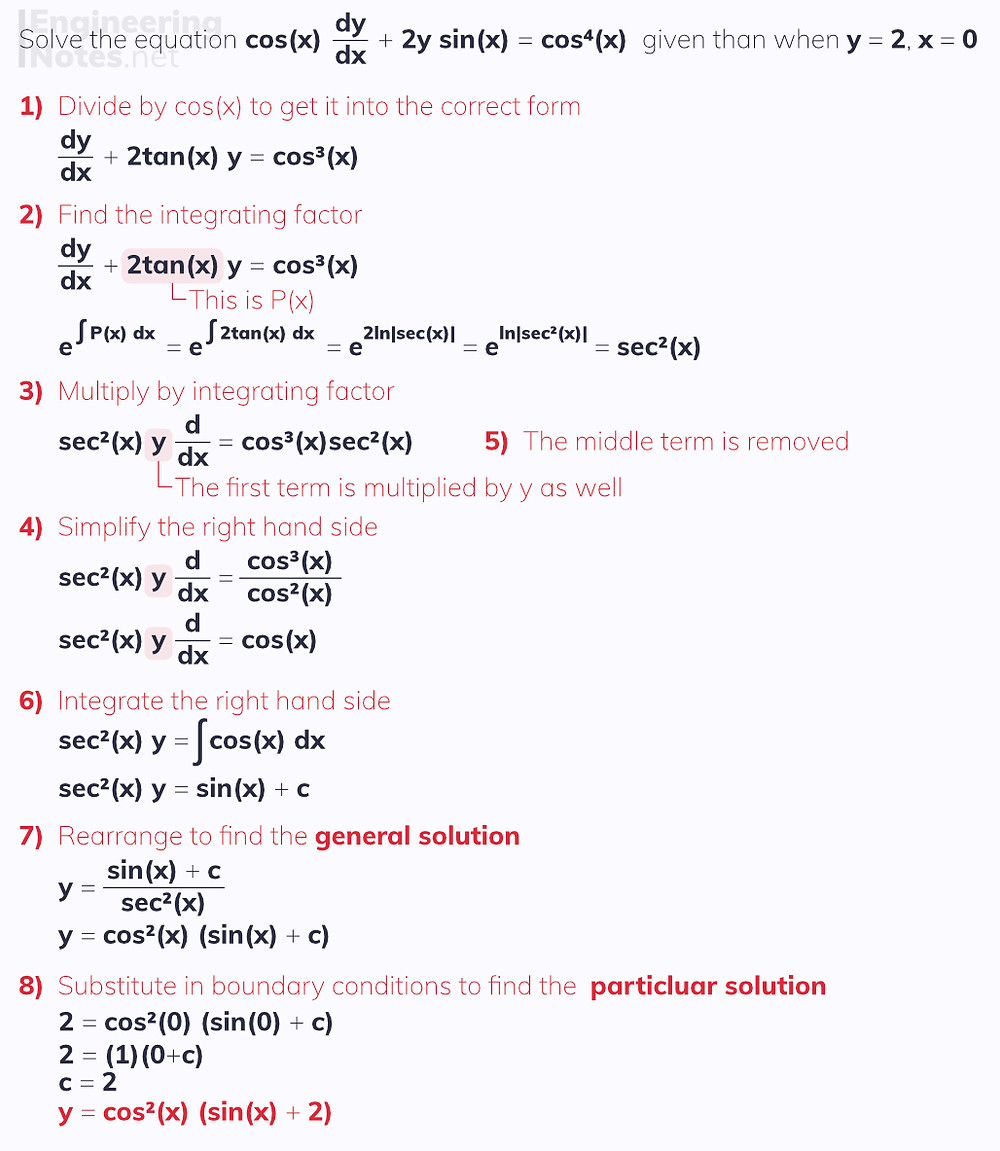#### Notes by Category University Engineering

Electronics*
Mathematics*
Mechanics & Stress Analysis*
Rate these notesNot a fanNot so goodGoodVery goodBrillRate these notes
• A-Level Further Maths

# Differential Equations

In single maths, first-order differential equations are the only ones looked at, and are solved by separating the variables:

When dy/dx = f(x) g(y), you can say ∫ 1/g(y) dy = ∫ f(x) dx
• Move all the y terms to the left where the dy is

• Move all the x terms to the right, including the dx

This allows you to integrate each side with respect to the variable on that side to solve the equation.

• You only need to add the ' +c ' to one side.Just like when integrating an indefinite function, the initial result is a general solution and could be anywhere along the y-axis (see section on indefinite integral functions above). To fond the particular solution, you need to know a coordinate point on the curve - sometimes this is called a boundary condition.

### Integrating Factor

This is not covered in single maths, but is an important method of solving first-order differential equations where x and terms are multiplied by one another in one of the terms:1. Rearrange to be in the form dy/dx + P(x)y = Q(x)

2. Find the integrating factor using the formula above

3. Multiply the dy/dx by the integrating factor and by y

4. Multiply the right hand side by the integrating factor & simplify (if you can)

5. The middle term, P(x)y disappears

6. Move the dx to the right hand side and integrate this side

7. Rearrange to make y the subject - this is the general solution

8. Find the particular solution by substituting in boundary conditions (if there are any)